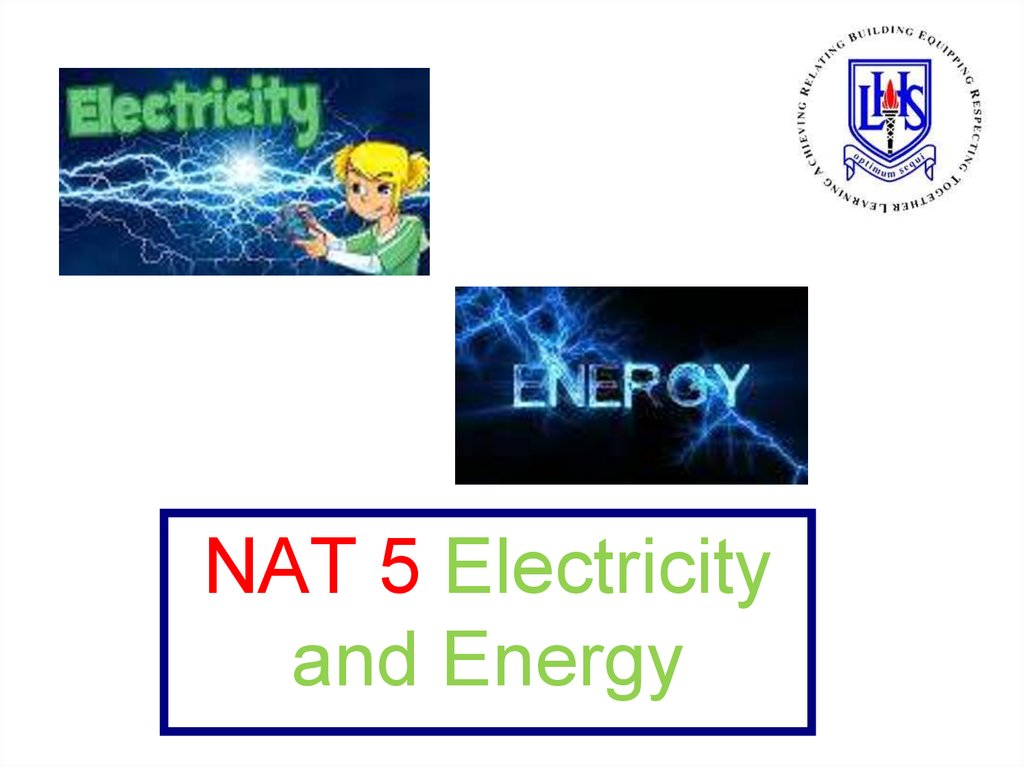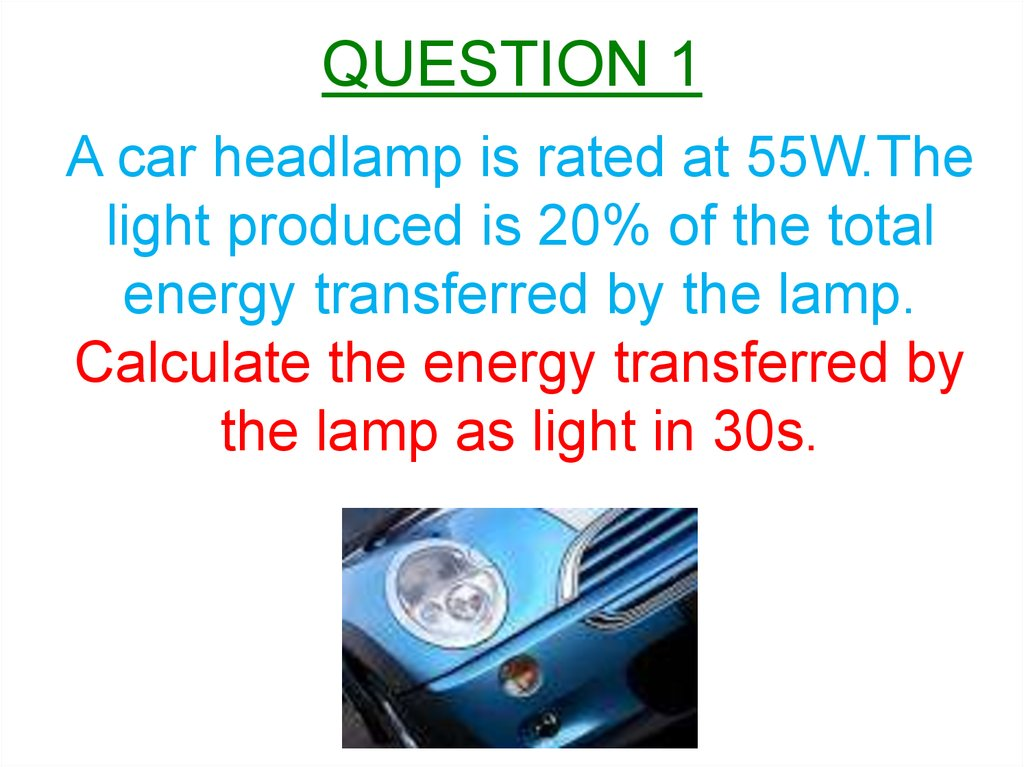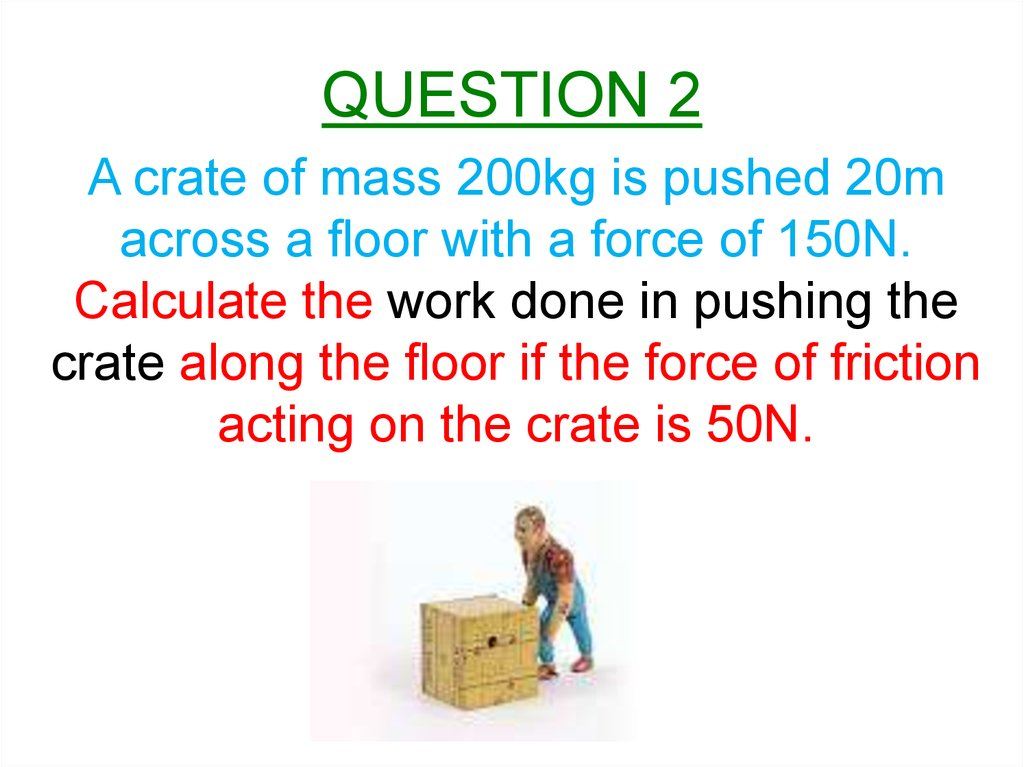Electricity and Energy

1.

NAT 5 Electricity
and Energy

2. QUESTION 1

A car headlamp is rated at 55W.The
light produced is 20% of the total
energy transferred by the lamp.
Calculate the energy transferred by
the lamp as light in 30s.

3. QUESTION 2

A crate of mass 200kg is pushed 20m
across a floor with a force of 150N.
Calculate the work done in pushing the
crate along the floor if the force of friction
acting on the crate is 50N.

4. QUESTION 3

An engine applies a force of 2000N to
move a lorry at a constant speed.
The lorry travels 100m in 16s.
Calculate the power developed by the engine.

5. QUESTION 4

An arrow of mass 150g is fired from a bow as
shown below. The archer pulls the string back
a distance of 0.50m and exerts a force of 300N
on the arrow as it is fired.
Calculate the maximum kinetic energy gained
by the arrow.

6. QUESTION 5

A power station has an efficiency of 40%.
The input power to the station is 1600MW.
Calculate the useful power output.

7. QUESTION 6

A person using the exercise bike below, pedals
against 300N of frictional forces applied to the
wheel of circumference 1.5m by the brake.
a) How much work is done against friction with one
turn of the wheel?
b) Calculate the average power produced if the wheel
turns 500 times in 5 minutes.

8. QUESTION 7

An electric motor raises a crate of mass 500kg
through a height of 12m in 8s.
Calculate or find:
a) Ep gained by the crate.
b) Minimum power rating of the electric motor.

9. QUESTION 8

A ski lift with a gondola of mass 2000kg travels to a
height of 540m from the base station.
a) Calculate the gain in gravitational potential energy
of the gondola.
b) Calculate the speed of the gondola if it has 36,000J
of kinetic energy at one stage in the journey.

10. QUESTION 9

The speed-time graph below shows the
motion of a 700kg car.
a) Calculate the kinetic energy at A.
b) State the work done in bringing the car to
a halt between B and C.

11. QUESTION 10

The graph below shows the speed-time graph of a
0.50kg object when it is involved in a collision.
Calculate the kinetic energy lost by the object when it
is in a collision between S and T.

12. QUESTION 11

A 0.02kg mass detaches from a thread before the vehicle is
released from rest and falls 0.80m to the floor.
Calculate or find:
a) Gravitational potential energy stored in the mass
before it fell.
b) Speed of the mass before it hits the floor.

13. QUESTION 12

A child of mass 50kg is playing on a water slide as shown
below.
Calculate or find:
a) Gravitational potential energy of the child at the top.
b) Length of the slide, if 20N of frictional force acts on the
child producing 1400J of heat energy.

14. QUESTION 13

The voltage of an electrical supply is a
measure of the

15. QUESTION 14

Which of the following statements are

16. QUESTION 15

A charge of 15C passes through a
resistor in 12s. The potential difference
across the resistor is 6V.
Calculate the power developed in the
resistor.

17. QUESTION 16

A rechargeable battery is labelled
2600mAh.
Calculate the charge stored in the
battery.

18. QUESTION 17

The current in an 8Ω resistor is 2A.
Calculate the charge passing through the
resistor in 40s.

19. QUESTION 18

Identify the circuit symbols X,Y and Z.

20. QUESTION 19

The mains voltage in the UK is 230V ac.
Which row in the table below shows the
peak voltage and the mains supply?

21. QUESTION 20

A student has two electrical power supplies.
One is an ac supply and the other is a dc supply.
Label which supply in the diagrams below are ac and dc
and describe them both in terms of electron flow.

22. QUESTION 21

A circuit is set up as shown below.
Which switch or switches must be closed to
light lamp L1 only?

23. QUESTION 22

Which of the following statements that an
LHS Physics student made are true?

24. QUESTION 23

A student is given a task of combining three
resistors from a pack containing:
30Ω, 50 Ω, 80 Ω, 120 Ω and 270 Ω.
Show by calculation which three resistors(In
series or parallel?) should be used to give
a) the largest combined resistance
b) the lowest combined resistance.

25. QUESTION 24

Which circuit below is used to find the
resistance of Resistor R2?

26. QUESTION 25

In the circuit below the resistance of the
variable resistor is increased.
Which row in the table shows the effect on the
ammeters and voltmeters?

27. QUESTION 26

Calculate the potential difference across
the 2Ω resistor in the circuit below.

28. QUESTION 27

Calculate the following from the circuit below:
a) Current through the resistor.
b) Power supplied to the resistor.

29. QUESTION 28

In the circuit below the current in the lamp is
1.5A and the reading on the voltmeter is 6V.
Calculate the power in the lamp.

30. QUESTION 29

Calculate the total resistance between X
and Y in the network below.

31. QUESTION 30

Calculate the following in the circuit below:
a) Total resistance between Y and Z.
b) Total resistance between W and X.
c) Voltage across the 2Ω resistor when the
current in the 4Ω resistor is 0.10A.

32. QUESTION 31

Calculate the following from the circuit below:
a) Total resistance in the circuit.
d) Power developed in the 2Ω series resistor.

33. QUESTION 32

Calculate or find the following from the rating
plate of an electric food mixer.
a) Resistance of the food mixer
b) Current passing through the food mixer.
c)The fuse required for the food mixer plug.

34. QUESTION 33

The graph below shows the temperature of a
substance over a period of time.
Which row in the table shows the sections of the
graph when the substance is changing state?

35. QUESTION 34

The specific latent heat of fusion of a
substance is the heat energy required to

36. QUESTION 35

100g of solid is heated by a 50W heater.
Calculate the specific latent heat of fusion
of the substance using the graph below.

37. QUESTION 36

A block of ice of mass 1.5kg is placed in a room
at 20°C. If the block of ice is at 0°C, then
calculate the minimum energy required to turn
the ice at 0°C into water at 20°C.

38. QUESTION 37

A sample of water at a temperature of 100°C
absorbs 23,000J of heat energy.
Calculate the mass of water turned into steam
at 100°C using the necessary information from
the data book.

39. QUESTION 38

A fridge/freezer has water and ice dispensers.
0.15kg of water flows into the freezer at 14°C and is
cooled to 0°C.
a) Calculate the heat energy removed when the water cools.
b) Calculate how much heat energy is released when 0.15kg of
water at 0°C changes to 0.15kg of ice at 0°C.

41. QUESTION 39 (Cont’d)

From the experiment and the data obtained above,
calculate or find:
a) Specific heat capacity of the water.
b) Power rating of the immersion heater.
c) How does the calculated reading in a) compare with
the accepted value in the data book?
d) How could the experiment be improved to provide a
specific heat capacity nearer the accepted value in the
data book?

42. QUESTION 40

On the planet Mercury the surface temperature at night
is -173°C and during the day it is 307°C.
A rock of mass 80kg lying on the surface of the planet
absorbs 3.46 x 107J of heat energy during the day.
a) Calculate the specific heat capacity of the rock.
b) Calculate how long it would take to release
3.46 x 107J of heat energy during the night if it is
released at 1500W.

43. QUESTION 41

A rectangular block of wood of mass 2000g has
dimensions of 2m x 1m x 0.1m.
Calculate the greatest pressure that the block
can exert when lying on a level surface.

44. QUESTION 42

The air pressure inside a passenger cabin of
an aircraft is 92,000Pa during a flight with the
pressure outside being 42,000Pa.
Calculate the resultant force on the cabin door
of area 3m2, caused by the difference in air
pressure.

45. QUESTION 43

Which of the following statements that an LHS
Physics student wrote in her notebook are true?

46. QUESTION 44

A liquid is heated from 16°C to 40°C.
What is the temperature rise in the Kelvin
Scale?

47. QUESTION 45

Which of the following graphs illustrates the correct
relationship between the pressure P and the volume
V of a fixed mass of gas at constant temperature?

48. QUESTION 46

A student uses the apparatus shown below to investigate the
relationship between the pressure and the volume of a fixed
mass of gas at constant temperature.
a) Using all of the data, establish the relationship between the
pressure and the volume of the gas.
b) Use the kinetic model to explain the change in pressure as
the volume of the gas decreases.

49. QUESTION 47

On a cold morning at 2°C the pressure of a car
tyre was 3x105Pa.
After a motorway run the temperature of air in
the tyre rose to 57°C, with the volume of the air
in the tyre staying constant.
Calculate the pressure of the tyre when its
temperature reached 57°C.

50. QUESTION 48

A balloon with a volume of 6.0m3 contains a
fixed mass of gas at a temperature of 300K and
a pressure of 2.0kPa.
The gas is heated to 600K and the pressure
drops to 1.0kPa.
Calculate the new volume of the balloon.

51. QUESTION 49

Oxygen is held inside a cylinder at a pressure
of 2.82x106Pa and temperature of 19°C.
Calculate the pressure of the gas in the
cylinder if it is moved to a storage room at 5°C.

52. QUESTION 50

The apparatus below was used to investigate the
relationship between the volume and temperature of
a fixed mass of gas at constant pressure.
A table of results was then taken and recorded.

53. QUESTION 50 (Cont’d)

A student investigates the relationship between the
volume and the temperature of a fixed mass of gas at
constant pressure.
a) Using all of the data, establish the relationship between
the temperature and volume for the trapped air.
b) Calculate the volume of the trapped air when the
temperature of the water is 65°C.
c) Use the Kinetic Model to explain the change in
volume as the temperature increases in the
experiment.

54. QUESTION 51

Which row in the table shows the symbols for an
LED and a NPN transistor.

55. QUESTION 52

Which of the following devices converts
heat energy into electrical energy?

56. QUESTION 53

In the voltage divider circuit below an LED is
connected in series with a resistor.
a) State the purpose of the resistor.
b) When lit, the LED has a voltage of 3.5V across it
and a current of 200mA passing through it.
Calculate the unknown resistance.

57. QUESTION 54

A light sensor circuit contains a LDR and a resistor as
shown below.
Using the table, calculate the voltage across the LDR
when the light sensor is covered.

58. QUESTION 55

The circuit below charges a rechargeable battery
during daylight hours.
a) State the energy transformation in the solar cell.
At a particular light level, the voltage generated by
the solar cell is 1.5V.
b) Calculate the voltage across the rechargeable
battery at this light level.

59. QUESTION 56

Answer the following questions using the circuit below
and the table provided.
a) Name component X.
b) State the purpose of component X in the circuit.
c) Explain how the circuit operates to sound the buzzer.
d) Describe a practical application for this circuit.

60. QUESTION 57

Answer the following questions from the circuit below.
a) Name component X.
b)Explain how the circuit works to switch the heater on.
c) What is the purpose of the variable resistor.
d) Give a possible practical application for this circuit.

61. QUESTION 58

Answer the following questions using the circuit below.
a) Name the components X and Z.
b) What happens to the resistance of the thermistor as the
temperature increases?
c)When the voltmeter reading reaches 1.8V the MOSFET
switches on. Explain how the circuit operates when the
temperature rises.
d) Why is component X used instead of a fixed resistance R?

62. QUESTION 59

Answer the following question using the circuit below.
a) Calculate the voltage across the LDR when its
resistance is 4kΩ.
b) Explain how this circuit operates when the light
level falls below a certain value.
c) Give a possible practical application for this circuit.

63. QUESTION 60

Answer the following questions from the circuit below.
a) What happens to the voltage at point X when
switch S is closed.
b) State two changes which could be made to the
circuit so that the time taken for the LED to come on
is reduced.